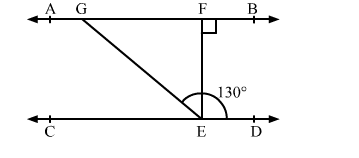# In the given figure, AB || CD and EF ⊥ AB. If EG is the transversal such that ∠GED = 130°, find ∠EGF.

Question:

In the given figure, AB || CD and EF ⊥ AB. If EG is the transversal such that ∠GED = 130°, find ∠EGF.Solution:

In the given figure, AB || CD and GE is the transversal.

∴ ∠GED + ∠EGF = 180°       (Sum of adjacent interior angles on the same side of the transversal is supplementary)

⇒ 130° + ∠EGF = 180°

⇒ ∠EGF = 180° − 130° = 50°

Thus, the measure of ∠EGF is 50°